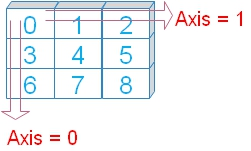# insert() : Adding at given position

We can add values at the given index position of a Numpy array by using append().
``numpy.insert(arr,obj, values, axis=None)``
`arr` : Values are added to the copy of this array.
`obj` : index or indices before which the value will be added.
`values` : Values to be added to arr. Must be of same shape of the arr ( if Axis is present ). If Axis is not specified then matching shape of arr is not prerequisite.
`axis` : (Optional) Direction in which the values to be appended. Arrays are flattened before insert() if axis is not given.

Note that there is no change to the original array.
``````import numpy as np
npr=np.array([5,8,3])
npr1=np.insert(npr,1,10) # adding value ( 10 ) at index position 1
print(npr) # [5 8 3]  , No change to original array
print(npr1)# [ 5 10  8  3], element added at 2nd position ``````
Adding value at end of the array by using len().
``````import numpy as np
npr=np.array([5,8,3])
npr1=np.insert(npr,len(npr),10) # adding at the end
print(npr)  # [5 8 3]  , No change to original array
print(npr1) # [ 5  8  3 10] , 10 added at the end``````
This is same as using append() to add value.

## Using 2-D array

if Axis is provided then adding array or values must have same shape of the original array. In case of any mismatch we will get this error message.
ValueError: could not broadcast input array from shape (1,5) into shape (1,4)
``````import numpy as np
npr=np.array([[0,1,2,4],
[3,4,5,6],
[6,7,8,9]])
#npr1=np.insert(npr,1,[10,11,12,13,14],axis=0) # valueError
npr1=np.insert(npr,1,[10,11,12,13],axis=0)
print(npr1)``````
Output
``````[[ 0  1  2  4]
[10 11 12 13]
[ 3  4  5  6]
[ 6  7  8  9]]``````

## Using axisAxis are the directions in rows and columns.

Axis 0 is the downward direction or in rows order.
Axis 1 is the horizontal direction or in column order.
``````import numpy as np
npr=np.array([[0,1,2,4],
[3,4,5,6],
[6,7,8,9]])
npr1=np.insert(npr,1,[10,11,12],axis=1)
print(npr1)``````
Output
``````[[ 0 10  1  2  4]
[ 3 11  4  5  6]
[ 6 12  7  8  9]]``````
We can specify multiple index positions to add values.
``````import numpy as np
npr=np.array([[0,1,2,4],
[3,4,5,6],
[6,7,8,9]])
npr1=np.insert(npr,[1,2,3],[10,11,12],axis=1)
print(npr1)``````
Output
``````[[ 0 10  1 11  2 12  4]
[ 3 10  4 11  5 12  6]
[ 6 10  7 11  8 12  9]]``````
Example
``````import numpy as np
npr=np.array([[0,1,2,4],
[3,4,5,6],
[6,7,8,9]])
npr1=np.insert(npr,[1,2,3],,axis=1)
print(npr1)``````
Output
``````[[ 0 99  1 99  2 99  4]
[ 3 99  4 99  5 99  6]
[ 6 99  7 99  8 99  9]]``````
Using different axis
``````import numpy as np
npr=np.array([[0,1,2,4],
[3,4,5,6],
[6,7,8,9]])
npr1=np.insert(npr,[1,2,3],,axis=0)
print(npr1)``````
Output
``````[[ 0  1  2  4]
[99 99 99 99]
[ 3  4  5  6]
[99 99 99 99]
[ 6  7  8  9]
[99 99 99 99]]``````
If axis is not given then the arrays are flattened before inserting.
``````import numpy as np
npr=np.array([[0,1,2,4],
[3,4,5,6],
[6,7,8,9]])
npr1=np.insert(npr,1,[10,11,12])
print(npr1)``````
Output
``[ 0 10 11 12  1  2  4  3  4  5  6  6  7  8  9]``
By using reshape() we can match the requirements of insert ( to match the shape ) and then use.
``````import numpy as np
npr=np.array([[0,1,2,4],[3,4,5,6],[6,7,8,9]])
npr1=np.array([10,11,12,13,21,22,23,24,31,32,34,35]) # different shape
#npr1=npr1.reshape(3,4)
npr1=npr1.reshape(npr.shape) # Match the shape of first array
npr2=np.insert(npr,2,npr1,axis=0)
print(npr2)``````
Output
``````[[ 0  1  2  4]
[ 3  4  5  6]
[10 11 12 13]
[21 22 23 24]
[31 32 34 35]
[ 6  7  8  9]]``````

## Example

Adding one element in each row. Note how the shape is matched.
``````import numpy as np
npr=np.array([[0,1,2,4],[3,4,5,6],[6,7,8,9]])
npr1=np.array([,,]) # One element for each row
npr2=np.insert(npr,[3,2,1],npr1,axis=1)
print(npr2)``````
Output
``````[[ 0 10  1 10  2 10  4]
[ 3 11  4 11  5 11  6]
[ 6 12  7 12  8 12  9]]``````

Subscribe to our YouTube Channel here

## Subscribe

* indicates required
Subscribe to plus2netplus2net.com